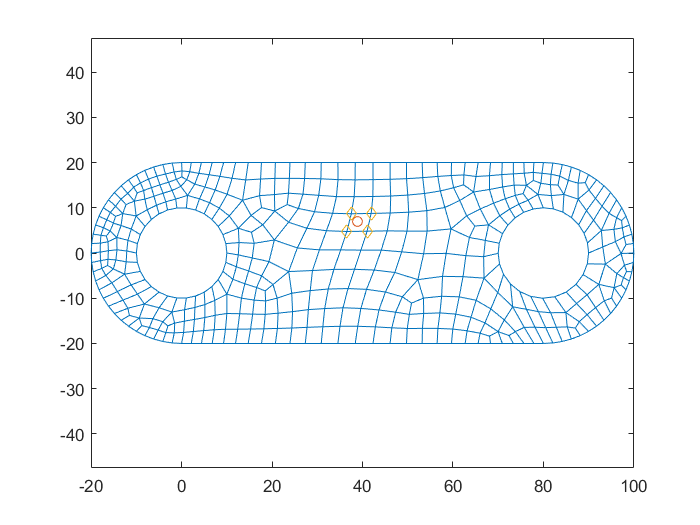# 2D Quadrilateral Interpolation: Barycentric Coordinates

## Interpolation on a general quadrilateral

When dealing with general quadrilaterals, the explicit expression of the shape functions is not easy to found. But we have the possibility of computing the value of such functions on a point p using the barycentric coordinates for quadrilaterals.
Similar to the triangular interpolation, now consider a point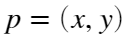, in a quadrilateral defined by four vertices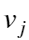numbered counterclokwise as usual(see figure). One can define the barycentric coordinades of this point which are equivalent to the value of the 2D shape functions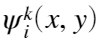defined for this quadrilateral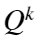:
satisfying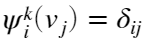.
This way, one can express any point using four coordinates (barycentric)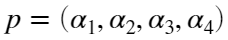. In particular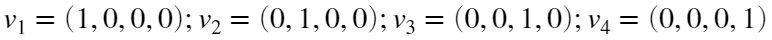.
The barycentric coordinates satisfy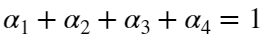. Moreover all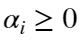unless the point is outside of the quadrilater.
Thus, the coordinates of the point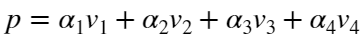.
To compute the values of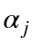we re-write the previous equation as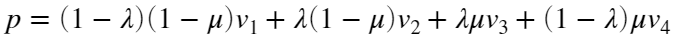.
where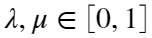Therefore,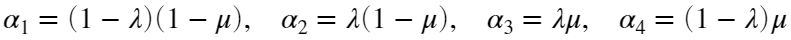Now to compute these two parameters, we rename the terms in the previous equation as: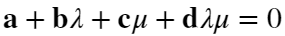where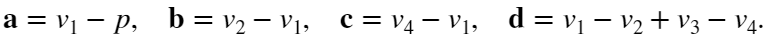Finally we apply Newton's method to obtain.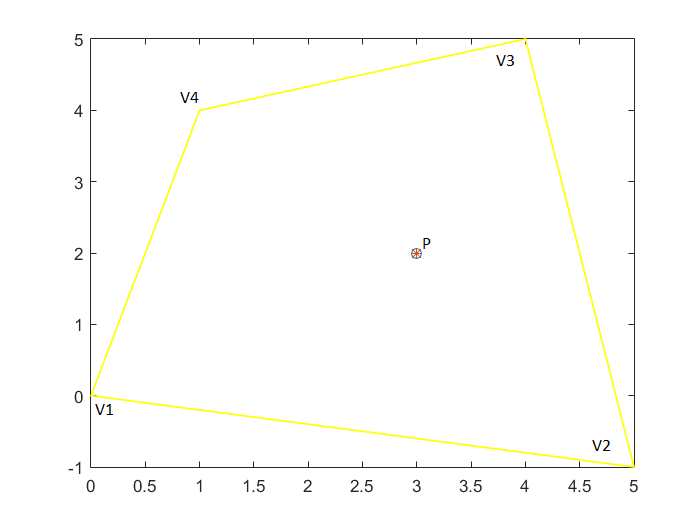## Compute Barycentric Coordinates on a general quadrilateral

Given a quadrilateral defined by vertices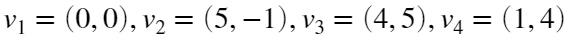, compute the barycentric coordinates of point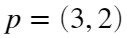.
Verify that.
% Data values
p=[3 2];
v1=[0 0];
v2=[5 -1];
v3=[4 5];
v4=[1 4];
% define the previous notation
a=(v1-p);
b=v2-v1;
c=v4-v1;
d=v1-v2-v4+v3;
% we use (x,y) instead of (lambda, mu) for easy code
% solution is supposed to have 0 <= x <=1 and 0 <= y <=1
x=0.5; y=0.5; %initial point
dx=0.1*ones(2,1);
iter=0;
tol=1.e-12;
while(norm(dx) > tol && iter < 20 )
%apply Newton-Rapson method to solve f(x,y)=0
f = a + b*x + c*y + d*x*y;
% Newton: x_{n+1} = x_n - (Df^-1)*f
% or equivalently denoting dx = x_{n+1}-x_n
% Newton: Df*dx=-f
Df(:,1)=(b + d*y); %df/dx
Df(:,2)=(c + d*x); %df/dy
bb=-f'; %independent term
dx=Df\bb;
x=x+dx(1);
y=y+dx(2);
iter=iter+1;
if (norm([x,y]) > 10), iter=20; end %non convergent: just to save time
end
if (iter < 20)
lambda=x;
mu=y;
%
alpha1=(1-mu)*(1-lambda);
alpha2=lambda*(1-mu);
alpha3=mu*lambda;
alpha4=(1-lambda)*mu;
alphas=[alpha1,alpha2,alpha3,alpha4]
else
alphas=[-1,-1,-1,-1]; %wrong values
end
alphas = 1×4
1.8750e-01 3.1250e-01 3.1250e-01 1.8750e-01
% obtained point
q=alpha1*v1+alpha2*v2+alpha3*v3+alpha4*v4;
p-q %we must recover the same point
ans = 1×2
0 0
% Plot the results
vertices=[v1,v2,v3,v4];
plotRectangle(v1,v2,v3,v4);
hold on;
plot(p(:,1),p(:,2),'o');
plot(q(:,1),q(:,2),'*');
hold off;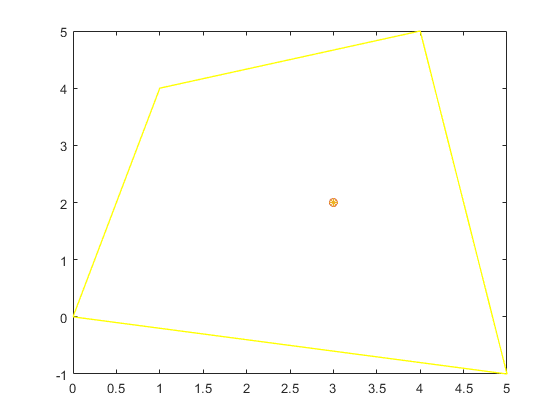## Exercise 1:

From the previous code implement the function baryCoordQuad (the Quadrilateral version of the baryCoord function).